# TARGET SBI EXAM 2018: QUANTITATIVE- QUADRATIC EQUATION-2

Dear Bankersdaily Aspirants,

Hope , Your preparation for SBI Clerk & PO exam 2018 is good . Since SBI Clerk & PO exam is a Two level which will be conducted in an online mode, Competition will be higher for this SBI Clerk & PO Exam and the most important thing , Current affairs section will be there in this exam. So aspirants have to concentrate in Four sections namely Aptitude, Reasoning, English, Current Affairs.

The person with high speed and very good accuracy only can crack this exam to taste the success. The candidates will be selected on the basis of performance in this online exam test. So the candidate with consistent hard work and regular practice will crack the exam very easily.

Exam: SBI  Clerk & PO Exam 2018

Timing: 7 minutes

Q.1)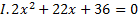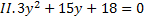a)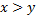b)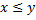c)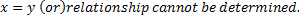d)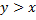e)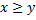Q.2)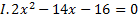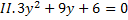a)b)c)d)e)Q.3)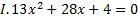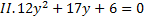a)b)c)d)e)Q.4)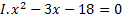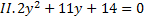a)b)c)d)e)Q.5)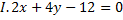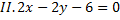a)b)c)d)e)D 6-10) Solve the equations and give the relationship between x and y.

a)b)c)d)e) x=y or Relationship can’t be determined

Q.6)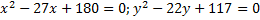Q.7)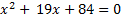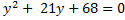Q.8)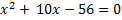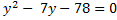Q.9)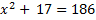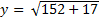Q.10)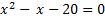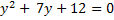Q.1) c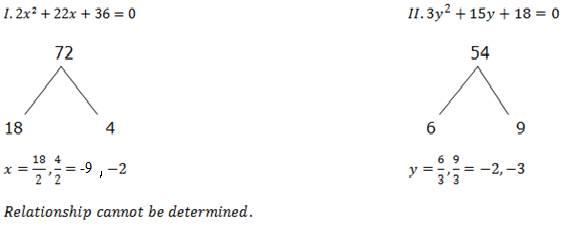Q.2) e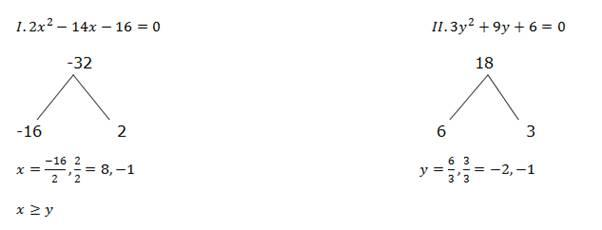Q.3) e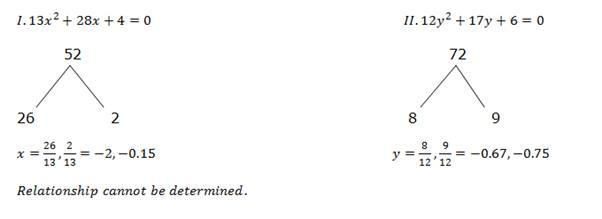Q.4) e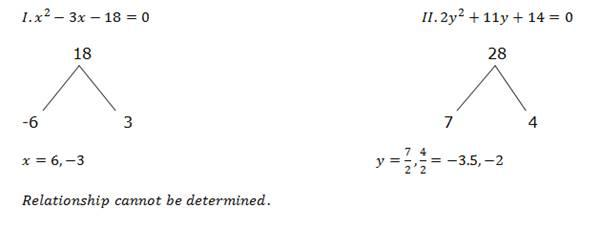Q.5) a

2x+4y-12=0——–1

2x-2y-6=0———2

Solve the above equation and we get X and Y value.

x=4, Y=1

Q.6)e

x^2-27x+180=0

x^2-12x-15x+180=0

(x-12)(x-15)

y^2-22y+117=0

(y-13)(y-9)

(y-13)(y-9)

y=13,9

Relationship can’t be determined.

Q.7)e

x^2+19x+84=(x+12)(x+7)

x=-12,-17

y^2+21y+68=(y+17)(y+4)

y=-17,-4

Relationship can’t be determined

Q.8)e

x^2+10x-56=(x+14)(x-4)

x=-14,4

y^2-7y-78=(y-13)(y+6)

y=13,-6

Relationship can’t be determined

Q.9)d

x^2+17=186

x^2=169

x=+(or)- 13

y=square root of (152+17)

y=square root of (169)

y=13

x ≤ y

Q.10)e

X^2-x-20=(x-5)(x+4)

x=5,-4

y^2+7y+12=(y+3)(y+4)

y=-3,-4

Relationship can’t be determined

For the other Sections in the Day 3 of the TARGET SBI PLANNER , please check the below given links.

Aspirants can also check the other topics from the SBI CLERK STUDY PLANNER from the link given below. DO bookmark the given link as all the posts will be updated in that page and aspirants can check the daily updates from this page or from the Homepage of Bankersdaily.